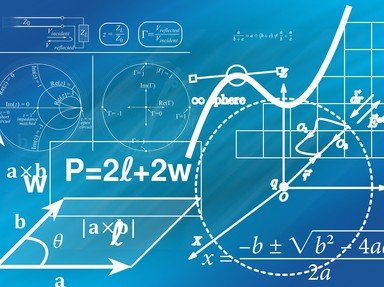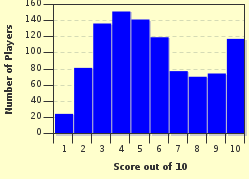FREE! Click here to Join FunTrivia. Thousands of games, quizzes, and lots more!# Euler's Number and Euler's Constant Quiz

### Many people think that Euler's number (2.718...) and the Euler's constant (0.5772...) refer to the same number, but they do not. This quiz tests your knowledge on these two beautiful mathematical constants. Enjoy!

A multiple-choice quiz by Matthew_07. Estimated time: 6 mins.

Author
Time
6 mins
Type
Multiple Choice
Quiz #
317,221
Updated
Dec 03 21
# Qns
10
Difficulty
Tough
Avg Score
5 / 10
Plays
3872
Last 3 plays: Bugnutz (10/10), Guest 35 (9/10), Guest 49 (5/10).
- -
Question 1 of 10
1. The famous Euler's number is also known as Napier's constant. A typical scientific calculator displays the numerical value of this mathematical constant up to 10 significant figures, namely 2.718281828. This figure is misleading because Euler's number is actually an IRRATIONAL number.

#### NEXT>

Question 2 of 10
2. Euler's number is denoted by the letter e. It is named after the Swiss mathematician, Leonhard Euler. This mathematical constant is also the base of the natural logarithm, as in log base e. Which of the following is the alternative notation for log base e? Hint

#### NEXT>

Question 3 of 10
3. Jacob Bernoulli, a Swiss mathematician defined Euler's number as the numerical value of the limit of (1 + 1/n)^n when n approaches infinity. In calculus, the definition of Euler's number is derived from the natural logarithm function. It states that e is simply the number such that log base e of the number e equals to what number? Hint

#### NEXT>

Question 4 of 10
4. The numerical value of e can be expressed in the following series: 1 + 1/1! + 1/2! + 1/3! + ... What is the name of this series? Hint

#### NEXT>

Question 5 of 10
5. Euler's number appears in Euler's equation, namely e^(i@) = cos@ + i x sin@, where i is the imaginary number (square root of -1) and @ is theta. Which of the following is the resulting Euler's identity if you substitute @ = pi (3.142...) into the original Euler's equation? (Hint: sin pi = 0 and cos pi = -1) Hint

#### NEXT>

Question 6 of 10
6. The Euler's constant, or the Euler-Mascheroni constant refers to the number 0.57721... It is used widely in the field of number theory and mathematical analysis. Which lowercase Greek letter denotes this Euler's constant? Hint

#### NEXT>

Question 7 of 10
7. The formal definition of the Euler's constant is given by the difference between a series (1/1 + 1/2 + 1/3 + ...+ 1/n) and the natural logarithm as n approaches infinity. What is the name of the series? Hint

#### NEXT>

Question 8 of 10
8. Which English mathematician, who was the mentor of the celebrated Indian mathematician, Srinivasa Ramanujan and also the author of the essay "A Mathematician's Apology" offered his position as the Savilian Professor of Geometry at the University of Oxford, England to anyone who could prove that the Euler's constant is irrational? Hint

#### NEXT>

Question 9 of 10
9. The famous definite integral of the function e^(-x) times (ln x)^2 with respect to x from 0 to infinity gives a value of 1.97811..., which is also equivalent to a^2 + (b^2)/6, where a and b are two distinct mathematical constants. What are a and b? Hint

#### NEXT>

Question 10 of 10
10. Let x = Euler's number (2.718...) and y = Euler's constant (0.5772...). The constant x^y is a very important mathematical constant that is used in the field of number theory. The value of x^y is closest to which of the following integers? Hint

 (Optional) Create a Free FunTrivia ID to save the points you are about to earn:Select a User ID:Choose a Password:Your Email:

Most Recent Scores
Dec 03 2023 : Bugnutz: 10/10
Dec 02 2023 : Guest 35: 9/10
Nov 28 2023 : Guest 49: 5/10
Nov 26 2023 : Galvani: 6/10
Nov 21 2023 : jonnowales: 6/10
Nov 19 2023 : rahul0: 7/10
Nov 12 2023 : Guest 136: 0/10
Oct 23 2023 : jazh2: 2/10
Oct 23 2023 : Djinn003: 2/10

Score DistributionQuiz Answer Key and Fun Facts
1. The famous Euler's number is also known as Napier's constant. A typical scientific calculator displays the numerical value of this mathematical constant up to 10 significant figures, namely 2.718281828. This figure is misleading because Euler's number is actually an IRRATIONAL number.

One might think that the number string 1828 repeats itself to give e = 2.7182818281828... If this is the case, then we can express e in fraction. However, this is NOT the case.

The actual value of e is 2.718281828459... It is both irrational (cannot be expressed as a fraction) and transcendental (not algebraic, namely not a root of polynomials with constant coefficients). Leonhard Euler himself proved that e is irrational.
2. Euler's number is denoted by the letter e. It is named after the Swiss mathematician, Leonhard Euler. This mathematical constant is also the base of the natural logarithm, as in log base e. Which of the following is the alternative notation for log base e?

Note that the first letter of the notation "ln" is the lowercase L and not the uppercase i. Let x be an unknown. Log base e x = ln x. An interesting property is e^(In x) = x.
3. Jacob Bernoulli, a Swiss mathematician defined Euler's number as the numerical value of the limit of (1 + 1/n)^n when n approaches infinity. In calculus, the definition of Euler's number is derived from the natural logarithm function. It states that e is simply the number such that log base e of the number e equals to what number?

For x greater than 1, the natural logarithm function, ln x refers to the area bounded by the hyperbola y = 1/x, x-axis, the vertical line x1 = 1 and x2 = x. When x2 = e, the area is ln e = 1. On the other hand, for x between 0 and 1, ln x is the negative of the area bounded by the hyperbola y = 1/x, x-axis, x1 = x and x2 = 1.
4. The numerical value of e can be expressed in the following series: 1 + 1/1! + 1/2! + 1/3! + ... What is the name of this series?

Define a function f(x) = e^x.

Notice that no matter how many times you differentiate the function f(x), you will get back the original function.

The general form of a Taylor series is the summation from n = 0 to infinity of f^(n)(a) x (x - a)^n / n!

When the neighborhood, a is chosen as 0, the Taylor series is called a MacLaurin series.
5. Euler's number appears in Euler's equation, namely e^(i@) = cos@ + i x sin@, where i is the imaginary number (square root of -1) and @ is theta. Which of the following is the resulting Euler's identity if you substitute @ = pi (3.142...) into the original Euler's equation? (Hint: sin pi = 0 and cos pi = -1)

Answer: e^(i x pi) + 1 = 0

e^(i@) = cos@ + i x sin@

Substitute @ = pi

e^(i x pi) = cos pi + i x sin pi
e^(i x pi) = -1 + i x 0
e^(i x pi) = -1 + 0
e^(i x pi) + 1 = 0

It is interesting to note that five fundamental mathematical constants appear in this Euler's identity, which are e (2.718...), pi (3.142...), i (imaginary number), 0 (additive identity) and 1 (multiplicative identity).
6. The Euler's constant, or the Euler-Mascheroni constant refers to the number 0.57721... It is used widely in the field of number theory and mathematical analysis. Which lowercase Greek letter denotes this Euler's constant?

The Swiss mathematician, Leonhard Euler first proposed this mathematical constant in 1735. In 1790, Lorenzo Mascheroni, an Italian mathematician published a paper discussing the same constant.
7. The formal definition of the Euler's constant is given by the difference between a series (1/1 + 1/2 + 1/3 + ...+ 1/n) and the natural logarithm as n approaches infinity. What is the name of the series?

The harmonic series itself (1/1 + 1/2 + 1/3 + ... ) is divergent. However, when the resulting numerical value is combined with the negative natural logarithm, -ln n, the series is convergent. As n approaches infinity, the series converges to the Euler's constant. The series converges very slowly to 0.55721...
8. Which English mathematician, who was the mentor of the celebrated Indian mathematician, Srinivasa Ramanujan and also the author of the essay "A Mathematician's Apology" offered his position as the Savilian Professor of Geometry at the University of Oxford, England to anyone who could prove that the Euler's constant is irrational?

G. H. Hardy, or his full name, Godfrey Harold Hardy was an English mathematician who was known for his work in the field of mathematical analysis and number theory.
9. The famous definite integral of the function e^(-x) times (ln x)^2 with respect to x from 0 to infinity gives a value of 1.97811..., which is also equivalent to a^2 + (b^2)/6, where a and b are two distinct mathematical constants. What are a and b?

Answer: a = the Euler's constant (0.5772...), b = pi (3.142...)

The integral was discovered by Whittaker and Watson in 1990. In fact, the Euler's constant appears in many other interesting integrals.
10. Let x = Euler's number (2.718...) and y = Euler's constant (0.5772...). The constant x^y is a very important mathematical constant that is used in the field of number theory. The value of x^y is closest to which of the following integers?

The value of x^y, or e^(gamma) = 1.78107... This constant appears in Mertens' third theorem, which is used to examine the density of prime numbers.
Source: Author Matthew_07

This quiz was reviewed by FunTrivia editor crisw before going online.
Any errors found in FunTrivia content are routinely corrected through our feedback system.
Related Quizzes
This quiz is part of series Commission Quizzes:

Quizzes written for Commissions: #1 Any title, #4 By the Numbers, #16 One-word title, #17 Black or white, #22: More or less, #61: Book titles, #62: Categories

1. Turn Your COIN into CASH Easier
2. Euler's Number and Euler's Constant Tough
3. Warriors Easier
4. The Bride Wore White Very Easy
5. Less Than Expected Average
6. Between the World and Me Easier
7. General Lee Speaking Very Easy

Also part of quiz list